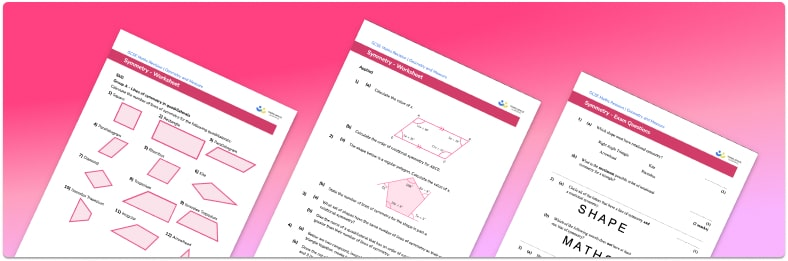# Similar Shapes Worksheet• Section 1 of the similar shapes worksheet contains 18 skills-based similar shapes questions, in 3 groups to support differentiation
• Section 2 contains 4 applied similar shapes questions with a mix of worded problems and deeper problem solving questions
• Section 3 contains 3 foundation and higher level GCSE exam style similar shapes questions
• Answers and a mark scheme for all similar shapes questions are provided
• Questions follow variation theory with plenty of opportunities for students to work independently at their own level
• All questions created by fully qualified expert secondary maths teachers
• Suitable for GCSE maths revision for AQA, OCR and Edexcel exam boards

• This field is for validation purposes and should be left unchanged.

You can unsubscribe at any time (each email we send will contain an easy way to unsubscribe). To find out more about how we use your data, see our privacy policy.

### Similar shapes at a glance

Similar shapes are shapes which are the same shape but may be different sizes and/or may have been flipped or rotated. The corresponding angles between the shapes are the same.

If two shapes are mathematically similar we can work out the scale factor of enlargement between the two shapes. For example, given two similar triangles, triangle ABC and triangle DEF, if we know that one side in triangle ABC is 4cm and the corresponding side in triangle DEF is 12cm then the scale factor is 124=3. We can then use this scale factor to work out missing lengths for the triangles.

As well as 2d shapes such as quadrilaterals and polygons, 3d shapes can be similar. We can find the scale factor between two 3d shapes in the same way as 2d shapes. Area scale factors are equal to the scale factor squared and volume scale factors are equal to the scale factor cubed.

Similarity is different to congruence in that for two shapes to be congruent they must be exactly the same shape and size whereas for two shapes to be similar they do not need to be the same size.

Looking forward, students can then progress to additional geometry worksheets, for example a 3D shapes worksheet or an.For more teaching and learning support on Geometry our GCSE maths lessons provide step by step support for all GCSE maths concepts.

## Do you have KS4 students who need more focused attention to succeed at GCSE?There will be students in your class who require individual attention to help them succeed in their maths GCSEs. In a class of 30, it’s not always easy to provide.

Help your students feel confident with exam-style questions and the strategies they’ll need to answer them correctly with our dedicated GCSE maths revision programme.

Lessons are selected to provide support where each student needs it most, and specially-trained GCSE maths tutors adapt the pitch and pace of each lesson. This ensures a personalised revision programme that raises grades and boosts confidence.

Find out more